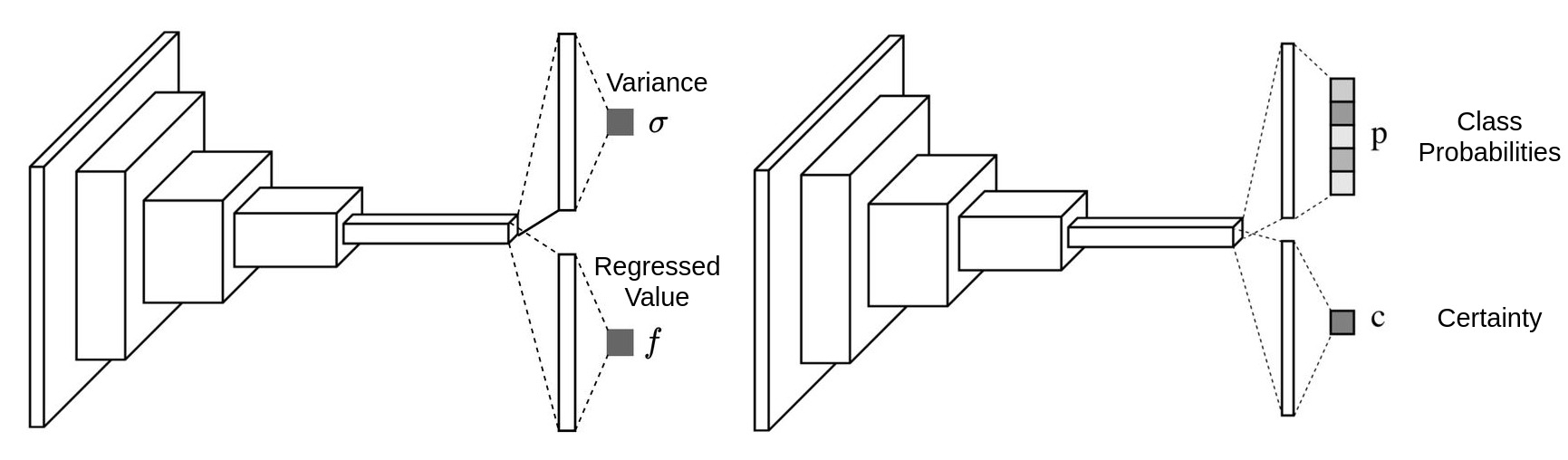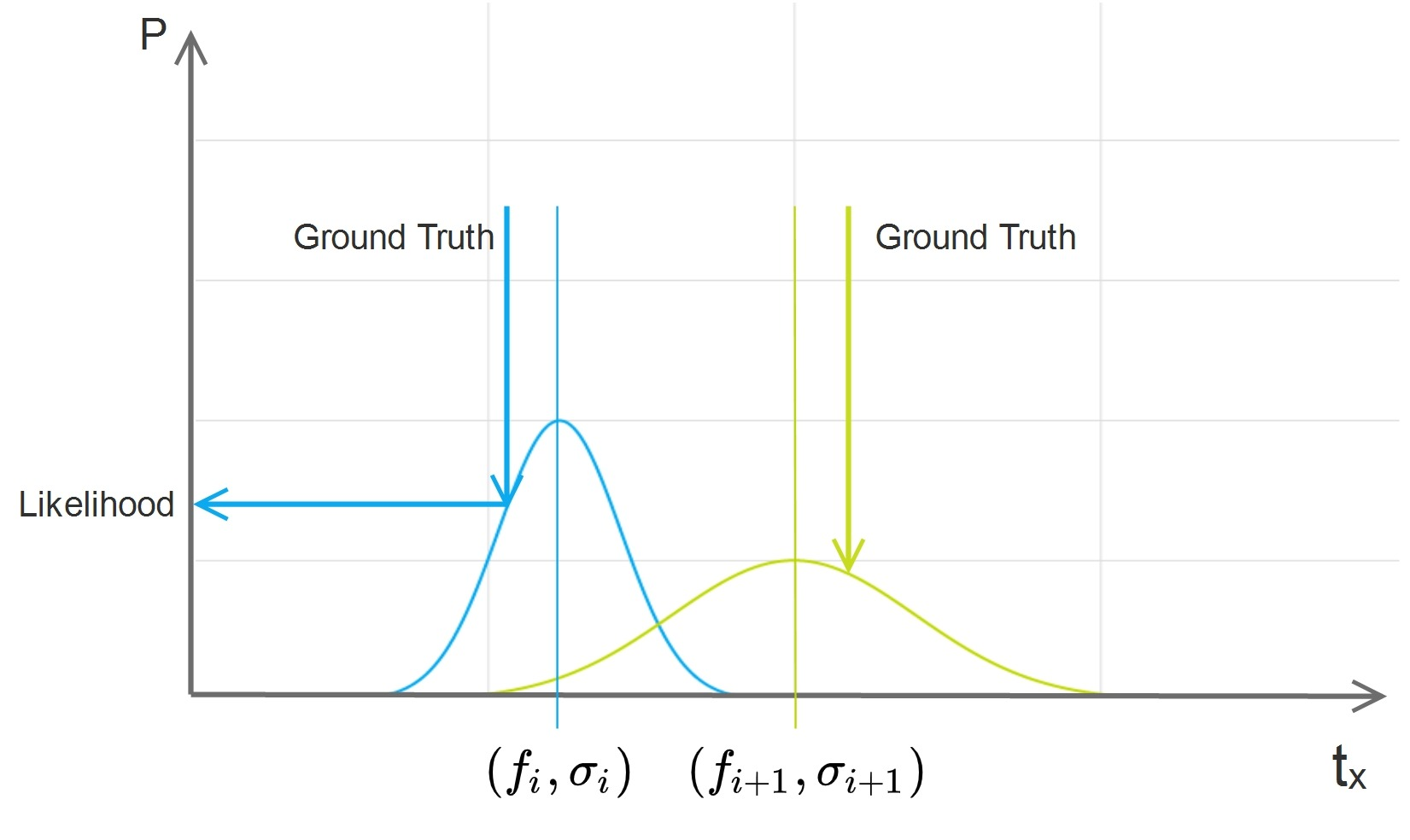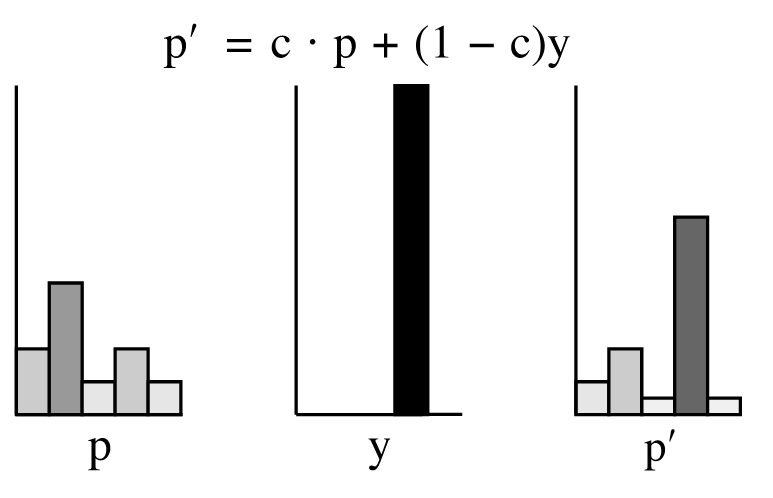# Uncertainty learning how to and why

### Uncertainty for classification and regression tasks

The ways of estimating uncertainty for both classification and regression tasks are similar. The uncertainty is considered as Variance ($$\sigma$$) for regression task and Certainty($$c$$) for classification task, it can be output as independent branch or just an additional channel alongside the other task:Once we get this additional output, we can then put it in a mathematical framework and form a loss function to make it learned automatically.

#### Uncertainty learning for regression tasks

Considering a regression task, it needs to regress the ground truth value $$y_i$$ and the regressed value from the network is $$f_i$$, usually we minimize the MSE loss to learn it:

$L_{mse}=\sum_{i=1}^N{(f_i-y_i)^2}$

Once the variance is added, what is really regressed is no longer a single value, but a complete Gaussian distribution defined by a mean $$f_i$$ and a variance $$\sigma_i$$, which leads to a maximum likelihood problem:From the example above, two pairs of value and variance are regressed, they have same MSE loss but different likelihood when variance is put into consideration. The likelihood with bigger variance is smaller.

The likelihood can be represented by a Gaussian distribution:

$Likelihood=\prod_{i=1}^N{\frac{1}{\sigma_i \sqrt{2\pi}}e^{-\frac{(f_i-y_i)^2}{2\sigma_i^2}}}$

We can then use negative log-likelihood as loss function to train it:

$L_{nll}=-log(Likelihood)=\sum_{i=1}^N{\frac{(f_i-y_i)^2}{2\sigma_i^2}}+log(\sigma_i)$

Once this loss function is minimized, the variance is learned. The bigger the variance, the flatter the Gaussian curve which leads to smaller likelihood.

#### Uncertainty learning for classification tasks

The logic for classification tasks is different. Let’s first take a look at a classification problem, which use cross-entropy as its loss function:

$L=-\sum_{i=1}^N{log(P_i)}$

Where $$N$$ is the number of training samples, $$P_i$$ is the output probability of the $$ith$$ sample’s ground truth label. Similar as the regression task, we output a certainty $$c_i$$.

The usual way of making use of this certainty value is computing a balanced $$P_i^{'}$$ by interpolating between the output and ground truth:Therefore the final loss function turns to be:

$L=-\sum_{i=1}^N{[log(c_i\cdot P_i+(1-c_i)\cdot y_i)}+log(c_i)]$

The reason behind is similar as the regression task, if the certainty is high, we rely more on the direct output; if the output is uncertain, we rely more on the ground truth, which means less gradients are propagated back and we learn less. In an extreme case, let’s assume the certainty is 0%, which means the output is not making any sense, we then use the ground truth as output and there will be 0 gradient propagated back. It is equivalent to ignoring this sample.

### Why uncertainty helps learning?

Uncertainty learning can not only provide more information, it can also improve the accuracy. This is one of the magic which uncertainty can play. But why?

The ideas behind can be understood in a straight forward way. The key of deep learning is teaching the neural network to learn correct things. This is the same as human being, when we are learning new knowledge, we should not follow the learning material completely but keep our critical thinking. Critical thinking means we should keep some doubts on the learning material and try to pick the correct things to learn from.

When we found out that the learning material is doubtful, we should then learn less from them, or even skip them completely.

Learning from good data is as important as skipping bad data. This is the key idea behind uncertainty learning. Once we learned to pick high quality data and skip low quality data, of course we can learn better. That’s why the overall accuracy will increase with the help of uncertainty learning.

### WangxinI am algorithm engineer focused in computer vision, I know it will be more elegant to shut up and show my code, but I simply can't stop myself learning and explaining new things ...

### What is the role of depth in neural networks?

#### Gaussian Yolo V3 - get 3.5 more mAP and uncertainty with 1% more computation

Published on December 01, 2019

#### Graph Optimization 4 - g2o introduction - GPS odometry

Published on November 18, 2018